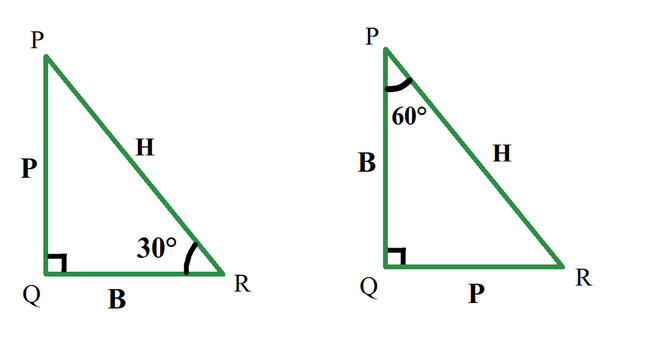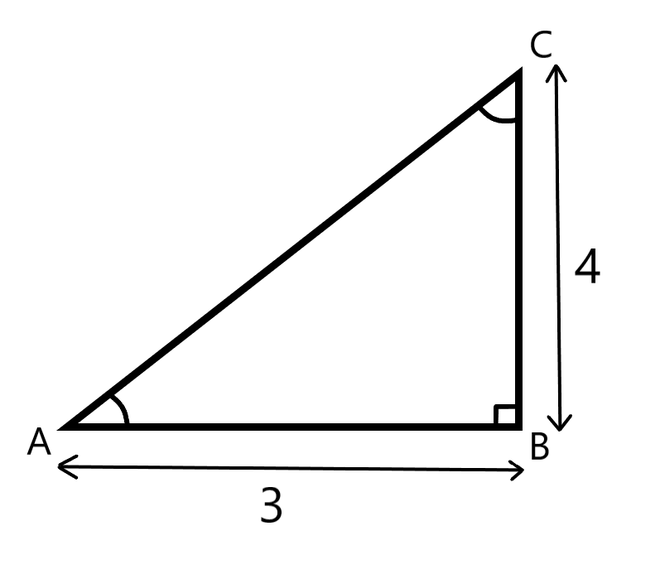Open in App
Not now

# If tan A = 4/3, then find the value of cos A

• Difficulty Level : Medium
• Last Updated : 20 Oct, 2021

Trigonometry is an important branch of mathematics, it deals with the relationship of sides with angles in a right-angle triangle. Trigonometry is important in physics also, it is used to find, the height of towers, the distance between stars, or in the Navigation systems. Trigonometry is predicated on the principle that “If two triangles have an equivalent set of angles then their sides are within the same ratio”. Side lengths are often different but side ratios are equivalent.

### Trigonometric Functions

Trigonometric functions or circular functions or trigonometric ratios show the relationship of angles and sides. These trigonometric ratios are obtained by taking ratios of sides. We have six trigonometric ratios Sin, Cos, Tan, Cosec, Sec, Cot.

• sin A = Perpendicular / Hypotenuse
• cos A = Base / Hypotenuse
• tan A = Perpendicular / Base
• cot A = Base / Perpendicular
• sec A = Hypotenuse / Base
• cosec A = Hypotenuse / Perpendicular

Here, A is that the angle opposite to the perpendicular side.

Let’s see what the Perpendicular, base, and hypotenuse of a right triangle are,

1. Perpendicular: The side ahead of the angle is perpendicular. In this case, the side in front of 30 deg is called it’s perpendicular.
2. Base: A base is one among the edges which contain an angle, except hypotenuse.
3. Hypotenuse: It is a side opposite to 90°. it is the largest side.

Note: Perpendicular and base changes as angle changes. During a triangle, a side is perpendicular for an angle, but an equivalent side may be a base for an additional angle, but the hypotenuse remains an equivalent because it’s a side opposite to angle 90°.In the above diagram for an equivalent triangle if considered angle 30° the perpendicular is that the side PQ, but if considered angle 60° the perpendicular is the side QR.

### If tan A = 4/3, then find the value of cos A

Solution:

Tan – The tan of an angle A is the ratio of lengths of perpendicular to the base.

Tan A = Perpendicular / Base

Cos – The cos of an angle A is the ratio of lengths of the base to the hypotenuse.

Cos A = Base / Hypotenuse

In a right triangle if tan A is 4/3 it looks as follows.tan A = 4/3

perpendicular / base = 4/3

Calculating hypotenuse –

H2 = P2 + B2

H2 = 42 + 32

H2 = 16 + 9

H2 = 25

H =5

Cos A = Base / hypotenuse

Cos A = 3/5

### Sample problem

Question 1: In a right angle triangle, angle A is 60°, and the base is 3m. Find the length of the hypotenuse.

Solution:

Given:  Base = 3m

Cos 60 = 1/2

B/H = 1/2

3/H = 1/2

H = 6

Question 2: In a right angle triangle, angle A is 30°, and the Hypotenuse is 3m. Find the length of the Base.

Solution:

Given:  Hypotenuse = 3m

Cos 30° = √3/2

B/H = √3/2

B/3 = √3/2

B = 3√3/2

Question 3: In a right angle triangle, for an angle A, the Perpendicular is 9√3m and the Base is 9m, find the angle A.

Solution:

Given:  perpendicular = 9√3m, Base = 9m.

Tan A = 9√3 / 9

Tan A = √3

Tan (60°) = √3

Angle A =  60°

My Personal Notes arrow_drop_up